# Congruent Triangle Proofs (Part 1)

When two triangles are said to be congruent, there is a correspondence that matches each angle to a congruent angle and each side to a congruent side.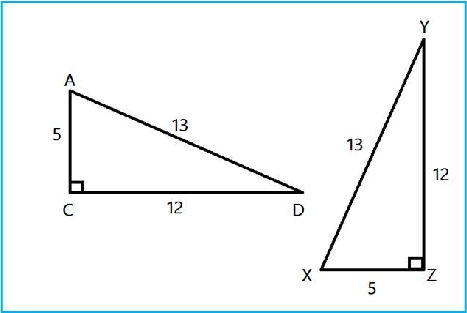Here, ΔADC is congruent to ΔXZY. So we write ΔADC ≅ ΔXZY.

What if we are not told that one triangle is congruent to another? There are several ways to tell if two triangles are congruent. Let's take a look at two of the methods.

Method 1: SSS (Side, Side, Side)

To use this method, we need to show that each side of one triangle is congruent to a side in the second triangle.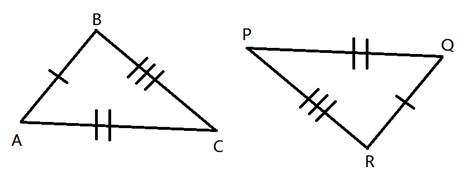In this example, side AB is congruent to side QR. Side AC is congruent to QP and side BC is congruent to side RP.
These two triangles are congruent because there are three pairs of congruent sides.

We use triangle congruence in mathematical proofs. Sometimes we will just need to show that two triangles are congruent. Other times, we will need to use the congruence to then show that some other fact about the triangles is also true.

Example #1: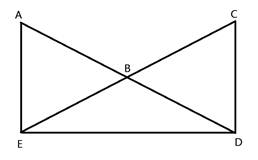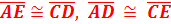Prove: < A ≅ < C

There are many triangles in this diagram. We are going to focus on only two of them. Here, we need to first show that ΔADE is congruent to ΔCED. We can then say that the corresponding parts of two congruent triangles are congruent in order to show that the angles are congruent.

Step 1: Set up two columns to show statements and reasons.

StatementsReasons

Step 2: Start to fill in the table with the given information.

StatementsReasons
1. AECD 1. Given
2. ADCE 2. Given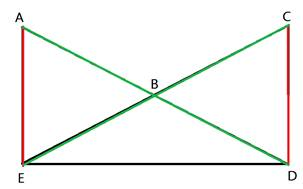Step 3: Look for any other given information that could help show that the two triangles are congruent. We have been given two pairs of congruent sides, so we can look for a third pair to show that these triangles are congruent. In this case, side DE is the same as side ED in the triangles. We call this the reflexive property

StatementsReasons
1. AECD 1. Given
2. ADCE 2. Given
3. EDDE 3. Reflexive Property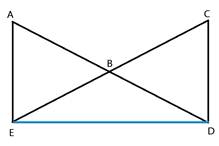Step 4: Show that the two triangles are congruent. We just showed that there are three pairs of congruent sides. Therefore, we used the SSS method.

StatementsReasons
1. AECD 1. Given
2. ADCE 2. Given
3. EDDE 3. Reflexive Property
4. ΔADE ≅ ΔCED 4. SSS

Step 5: Now that the two triangles are congruent, we can say that the corresponding side and corresponding angles are congruent. For the reason, we simplify this by just writing CPCTC which stands for "Corresponding parts of congruent triangles are congruent."

StatementsReasons
1. AECD 1. Given
2. ADCE 2. Given
3. EDDE 3. Reflexive Property
4. ΔADE ≅ ΔCED 4. SSS
5. < A ≅ < C 6. CPCTC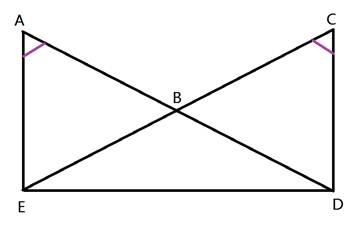So by first showing that two triangles were congruent because they had three sets of congruent corresponding sides, we can then show that the corresponding angles are also congruent.

 Related Links: Math Geometry Triangles Congruent Triangles

To link to this Congruent Triangle Proofs (Part 1) page, copy the following code to your site: